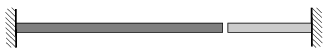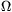Mathematical and Physical Journal
for High Schools
Issued by the MATFUND Foundation
 Already signed up? New to KöMaL?

# KöMaL Problems in Physics, September 2011

Show/hide problems of signs:## Problems with sign 'M'

Deadline expired on October 10, 2011.

M. 316. Measure how the number of revolutions of the electric motor of a toy depends on the input voltage. (The number of revolutions can be measured for example with a hand made stroboscope).

(6 pont)

statistics## Problems with sign 'P'

Deadline expired on October 10, 2011.

P. 4359. The figure shows the velocity-time graph of a lift in a mine. To what depth does the lift goes down?(3 pont)

solution (in Hungarian), statistics

P. 4360. What can an observer on the Moon experience, when the eclipse of the Moon can be seen from the Earth?

(3 pont)

solution (in Hungarian), statistics

P. 4361. A car travelled at a speed of, when a pedestrian stepped down from the sidewalk. The driver braked suddenly and the accident was avoided. The braking-distance was 6.25 m.

a) The car in the next lane could only stop in a distance of 9 m. What was the speed of this car if the accelerations of the two cars were the same.

b) What were the braking times of the two cars? c) What is the ratio of their initial kinetic energies if their mass is the same?

(4 pont)

solution (in Hungarian), statistics

P. 4362. 10 cm from a tap the diameter of the narrow water stream, flowing from the tap, is 2 mm. How long does it take to fill a half-litre mug?

(4 pont)

solution (in Hungarian), statistics

P. 4363. A simple heating system (in which there is no pump) can be the following: two tubes connect the boiler in the cellar and the radiator in the room above, allowing the water to flow in a closed path. One of the tubes connect the bottom of the boiler with the bottom of the radiator and the other connects the top of the radiator and the top of the boiler. In which direction and why will the water flow if the water is heated in the boiler?

(3 pont)

solution (in Hungarian), statistics

P. 4364. The figure shows two rods, a copper one and an aluminum one, which comes out of a wall. At a temperature of 20 oC the length of the copper rod is 2 m, and the length of the aluminum one is 1 m. The gap between them is 1.3 mm.

a) At what temperature will the gap disappear?

b) What is the size of the gap at a temperature of 0 oC?(4 pont)

solution (in Hungarian), statistics

P. 4365. In a container there is a sample of air at a pressure of 5.105 Pa, and at a temperature of 350 K. The air is compressed at constant pressure to 4/5 of its original volume, while a 1000 J work is done.

a) What will the temperature of the air be?

b) What is the change in the internal energy of the sample of air?

c) What is the density of the compressed air?

(4 pont)

solution (in Hungarian), statistics

P. 4366. The area of the bottom of a cylinder-shaped vessel is 78,5 cm2. The height of the water coloumn in it at a temperature of 18 oC is 15 cm. Then the temperature of both the vessel and the water is increased to 48 oC.

a) Will the hydrostatic pressure exerted to the bottom of the vessel increase or decrease? What percent is the change?

b) Will the gravitational potential energy of the water, with respect to the bottom of the vessel, increase or decrease? What percent is the change? (Neglect the evaporation of water!)

(5 pont)

solution (in Hungarian), statistics

P. 4367. An easily moveable, airproof piston of negligible mass closes a cylinder shaped container, made from some good heat-conducting material. The area of the base of the cylinder is 1,0 dm2. In the cylinder, below the piston, there is 2 ml water at room temperure, and a very little air. The cylinder is immersed into some liquid, the temperature of which is 100 oC, and is kept in it for a long time. What happens? At what height will the piston be at rest?

(5 pont)

solution (in Hungarian), statistics

P. 4368. Two resistors of resistances 1 kand 4 kare connected in series to a 220 V voltage supply of negligible internal resistance. Across the 4 kresistor a moving coil voltmeter reads 147 V. What would be the reading on this voltmeter if it was connected to measure the voltage across the other resistor?

(4 pont)

solution (in Hungarian), statistics

P. 4369. A pointlike light-source is moving parallel to the principal axis of a converging lens of focal length f at a distance of f/4 from the principal axis. How far is the light-source from the lens at that moment when its speed is equal to the speed of the image of the light-source?

(6 pont)

solution (in Hungarian), statistics